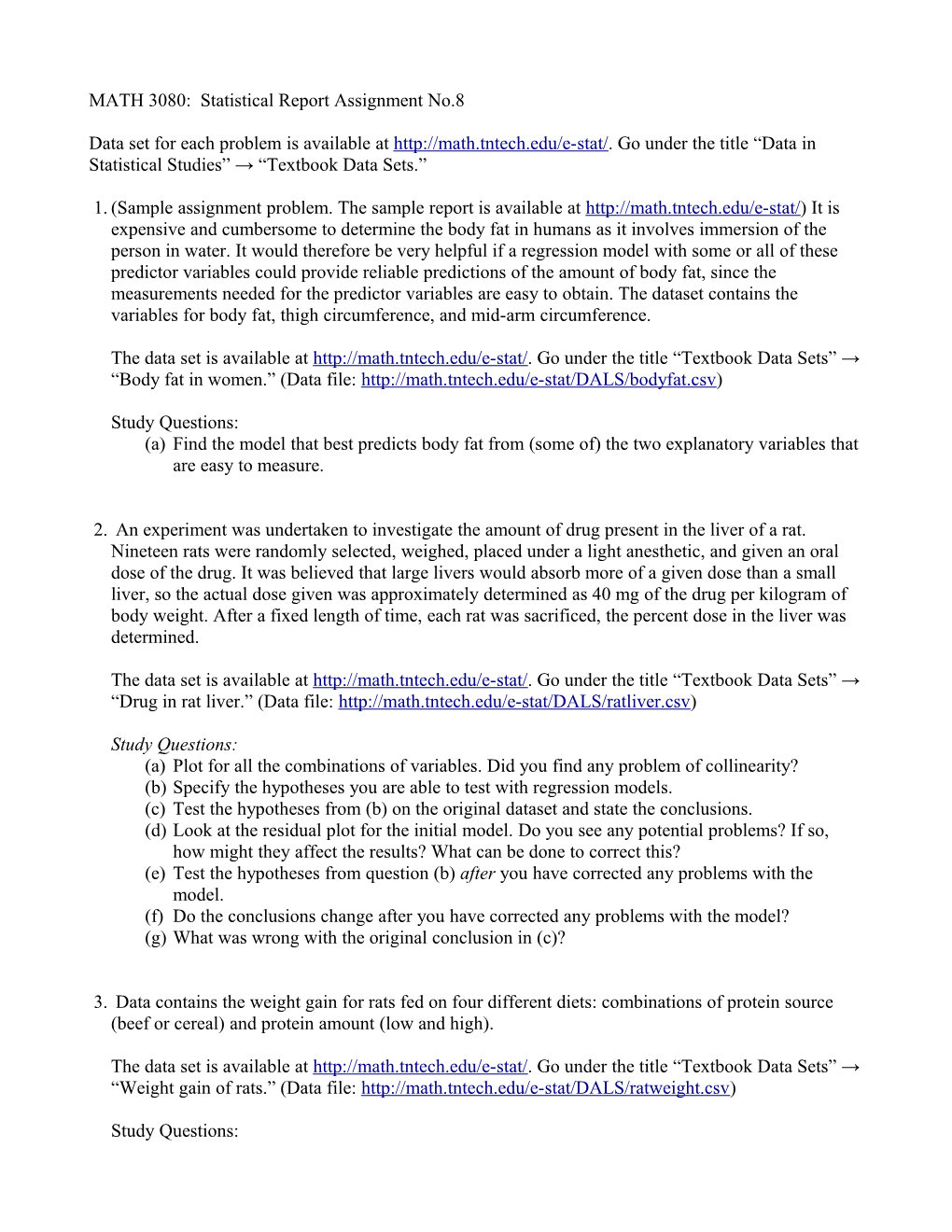# MATH 3080: Statistical Report Assignment No.8MATH 3080: Statistical Report Assignment No.8

Data set for each problem is available at Go under the title “Data in Statistical Studies” → “Textbook Data Sets.”

1.(Sample assignment problem. The sample report is available at is expensive and cumbersome to determine the body fat in humans as it involves immersion of the person in water. It would therefore be very helpful if a regression model with some or all of these predictor variables could provide reliable predictions of the amount of body fat, since the measurements needed for the predictor variables are easy to obtain. The dataset contains the variables for body fat, thigh circumference, and mid-arm circumference.

The data set is available at Go under the title “Textbook Data Sets” → “Body fat in women.” (Data file:

Study Questions:

(a)Find the model that best predicts body fat from (some of) the two explanatory variables that are easy to measure.

2. An experiment was undertaken to investigate the amount of drug present in the liver of a rat. Nineteen rats were randomly selected, weighed, placed under a light anesthetic, and given an oral dose of the drug. It was believed that large livers would absorb more of a given dose than a small liver, so the actual dose given was approximately determined as 40 mg of the drug per kilogram of body weight. After a fixed length of time, each rat was sacrificed, the percent dose in the liver was determined.

The data set is available at Go under the title “Textbook Data Sets” → “Drug in rat liver.” (Data file:

Study Questions:

(a)Plot for all the combinations of variables. Did you find any problem of collinearity?

(b)Specify the hypotheses you are able to test with regression models.

(c)Test the hypotheses from (b) on the original dataset and state the conclusions.

(d)Look at the residual plot for the initial model. Do you see any potential problems? If so, how might they affect the results? What can be done to correct this?

(e)Test the hypotheses from question (b)after you have corrected any problems with the model.

(f)Do the conclusions change after you have corrected any problems with the model?

(g)What was wrong with the original conclusion in (c)?

3. Data contains the weight gain for rats fed on four different diets: combinations of protein source (beef or cereal) and protein amount (low and high).

The data set is available at Go under the title “Textbook Data Sets” → “Weight gain of rats.” (Data file:

Study Questions:

(a)Specify a statistical model where weight gain is a function of protein source and protein amount. Identify the effect of treatment, and the effect of block. Justify the model.

(b)State the hypotheses you can test in this model.

(c)Test the hypotheses and state the conclusion. In particular, the investigators are interested in quantifying the differences found between the two protein sources as well as the differences found between the two protein amounts.